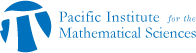## Discrete Math Seminar: Ruiyuan Chen

• Date: 11/08/2011
• Time: 16:00
Lecturer(s):
Ruiyuan (Ronnie) Chen
Location:

University of British Columbia

Topic:

Forbidden Submatrices

Description:

Abstract:We consider an extremal problem arising from forbidding a submatrix. Let F be a given (0,1)-matrix. Let avoid(m,F) denote the set of m-rowed (0,1)-matrices with no repeated columns and no submatrix F. Here we are concerned with row and column order. Let f(m,F) denote the maximum number of columns among all matrices in avoid(m,F). A conjecture of Anstee, Frankl, Furedi and Pach is that if F has k row, then there is a constant c(F) so that f(m,F)<c(F)m^k. We make progress in the case k=2 using an `amortized' analysis. This represents joint work with Richard Anstee and Attila Sali.

Other Information:

Location: MATX 1102